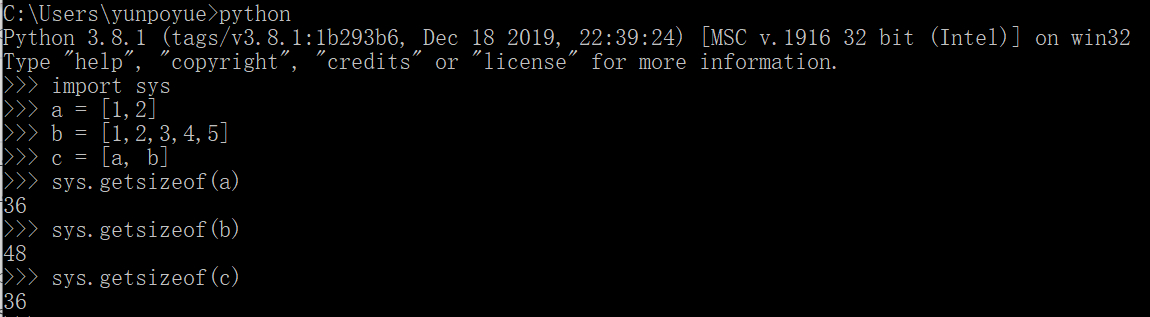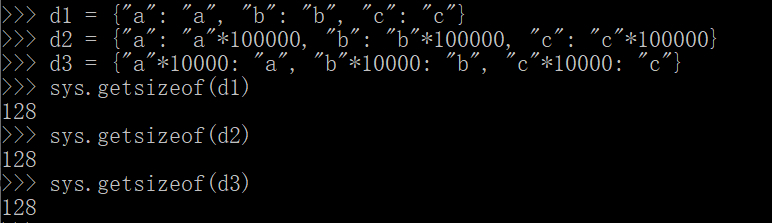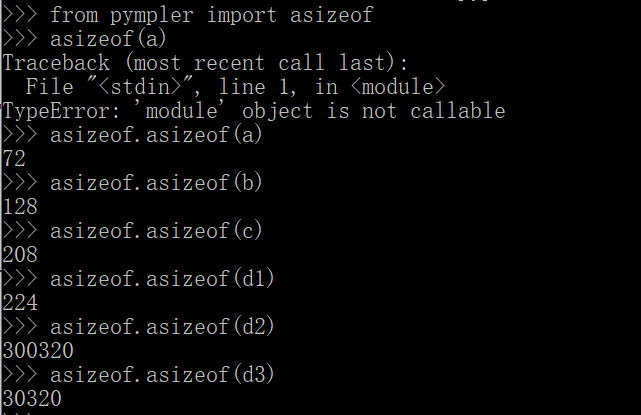## Python在计算内存时应该注意的问题？ 顶原荐

• 该方法用于获取一个对象的字节大小（bytes）
• 它只计算直接占用的内存，而不计算对象内所引用对象的内存

## 1、计算的是什么？## 2、“浅计算”与其它问题

“浅计算”不关心真实的对象，所以其计算结果只是一个假象。这是一个值得注意的问题，但是注意到这点还不够，我们还可以发散地思考如下的问题：

• “浅计算”方法的底层实现是怎样的？
• 为什么 getsizeof() 会采用“浅计算”的方法？

``````/*longobject.c*/

static Py_ssize_t
int___sizeof___impl(PyObject *self)
{
Py_ssize_t res;

res = offsetof(PyLongObject, ob_digit) + Py_ABS(Py_SIZE(self))*sizeof(digit);
return res;
}
``````

• 字节增大：int 类型在 C 语言中只占到 4 个字节，但是在 Python 中，int 其实是被封装成了一个对象，所以在计算其大小时，会包含对象结构体的大小。在 32 位解释器中，getsizeof(1) 的结果是 14 个字节，比数字本身的 4 字节增大了。
• 字节减少：对于相对复杂的对象，例如列表和字典，这套计算机制由于没有累加内部元素的占用量，就会出现比真实占用内存小的结果。

## 3、“深计算”与其它问题

• 是否存在“深计算”的方法/实现方案？
• 实现“深计算”时应该注意什么？

Stackoverflow 网站上有个年代久远的问题“How do I determine the size of an object in Python?” ，实际上问的就是如何实现“深计算”的问题。``````64
118
190
206
300281
30281
``````

pysize 项目很简单，只有一个核心方法：

``````def get_size(obj, seen=None):
"""Recursively finds size of objects in bytes"""
size = sys.getsizeof(obj)
if seen is None:
seen = set()
obj_id = id(obj)
if obj_id in seen:
return 0
# Important mark as seen *before* entering recursion to gracefully handle
# self-referential objects
if hasattr(obj, '__dict__'):
for cls in obj.__class__.__mro__:
if '__dict__' in cls.__dict__:
d = cls.__dict__['__dict__']
if inspect.isgetsetdescriptor(d) or inspect.ismemberdescriptor(d):
size += get_size(obj.__dict__, seen)
break
if isinstance(obj, dict):
size += sum((get_size(v, seen) for v in obj.values()))
size += sum((get_size(k, seen) for k in obj.keys()))
elif hasattr(obj, '__iter__') and not isinstance(obj, (str, bytes, bytearray)):
size += sum((get_size(i, seen) for i in obj))

if hasattr(obj, '__slots__'): # can have __slots__ with __dict__
size += sum(get_size(getattr(obj, s), seen) for s in obj.__slots__ if hasattr(obj, s))

return size
``````

``````    def asizeof(self, *objs, **opts):
'''Return the combined size of the given objects
(with modified options, see method **set**).
'''
if opts:
self.set(**opts)
self.exclude_refs(*objs)  # skip refs to objs
return sum(self._sizer(o, 0, 0, None) for o in objs)
``````

``````    def _sizer(self, obj, pid, deep, sized):  # MCCABE 19
'''Size an object, recursively.
'''
s, f, i = 0, 0, id(obj)
if i not in self._seen:
self._seen[i] = 1
elif deep or self._seen[i]:
# skip obj if seen before
# or if ref of a given obj
self._seen.again(i)
if sized:
s = sized(s, f, name=self._nameof(obj))
self.exclude_objs(s)
return s  # zero
else:  # deep == seen[i] == 0
self._seen.again(i)
try:
k, rs = _objkey(obj), []
if k in self._excl_d:
self._excl_d[k] += 1
else:
v = _typedefs.get(k, None)
if not v:  # new typedef
_typedefs[k] = v = _typedef(obj, derive=self._derive_,
frames=self._frames_,
infer=self._infer_)
if (v.both or self._code_) and v.kind is not self._ign_d:
# 猫注：这里计算 flat size
s = f = v.flat(obj, self._mask)  # flat size
if self._profile:
# profile based on *flat* size
self._prof(k).update(obj, s)
# recurse, but not for nested modules
if v.refs and deep &lt; self._limit_ \
and not (deep and ismodule(obj)):
z, d = self._sizer, deep + 1
if sized and deep &lt; self._detail_:
# use named referents
self.exclude_objs(rs)
for o in v.refs(obj, True):
if isinstance(o, _NamedRef):
r = z(o.ref, i, d, sized)
r.name = o.name
else:
r = z(o, i, d, sized)
r.name = self._nameof(o)
rs.append(r)
s += r.size
else:  # just size and accumulate
for o in v.refs(obj, False):
# 猫注：这里递归计算 item size
s += z(o, i, d, None)
# deepest recursion reached
if self._depth &lt; d:
self._depth = d
if self._stats_ and s &gt; self._above_ &gt; 0:
# rank based on *total* size
self._rank(k, obj, s, deep, pid)
except RuntimeError:  # XXX RecursionLimitExceeded:
self._missed += 1
if not deep:
self._total += s  # accumulate
if sized:
s = sized(s, f, name=self._nameof(obj), refs=rs)
self.exclude_objs(s)
return s
``````

``````    def flat(self, obj, mask=0):
'''Return the aligned flat size.
'''
s = self.base
if self.leng and self.item &gt; 0:  # include items
s += self.leng(obj) * self.item
# workaround sys.getsizeof (and numpy?) bug ... some
# types are incorrectly sized in some Python versions
# (note, isinstance(obj, ()) == False)
# 猫注：不可 sys.getsizeof 的，则用上面逻辑，可以的，则用下面逻辑
if not isinstance(obj, _getsizeof_excls):
s = _getsizeof(obj, s)
return s
``````

## 4、小结

getsizeof() 方法的问题是显而易见的，我创造了一个“浅计算”概念给它。这个概念借鉴自 copy() 方法的“浅拷贝”，同时对应于 deepcopy() “深拷贝”，我们还能推理出一个“深计算”。

Python 官方团队当然也知道 getsizeof() 方法的局限性，他们甚至在文档中加了一个链接 ，指向了一份实现深计算的示例代码。那份代码比 pysize 还要简单（没有考虑类对象的情况）。

### 相关链接

Python 内存分配时的小秘密：https://dwz.cn/AoSdCZfo

Python中对象的内存使用(一)：https://dwz.cn/SXGtXklz

 https://code.activestate.com/recipes/577504### 评论(0)

python学习03.02：Python数值类型（整形、浮点型和复数）及其用法

2019/10/07
40
0
Python之 is 和 == 的区别 字符串的intern机制

2018/04/03
49
3
[Python源码学习]之内存管理

Python 的内存管理架构（Objects/obmalloc.c）： 0. C语言库函数提供的接口 1. PyMem_*家族，是对 C中的 malloc、realloc和free 简单的封装，提供底层的控制接口。 2. PyObject_* 家族，高级...

2012/05/08
358
0
python多线程和多进程——python并行编程实验

2019/05/29
0
0

2019/10/21
0
0

IDEA Tomcat 日志乱码解决汇总

IDEA Tomcat 日志乱码解决汇总 第三种没解决，第二种配置后解决了 https://blog.csdn.net/sinat_32404247/article/details/90287700...

18
0
C/C++内存检查

chuqq

27
0
【附录】1、tapd在公司敏捷开发中的运用

27
0
Android 上显示 PDF 文件

22
0